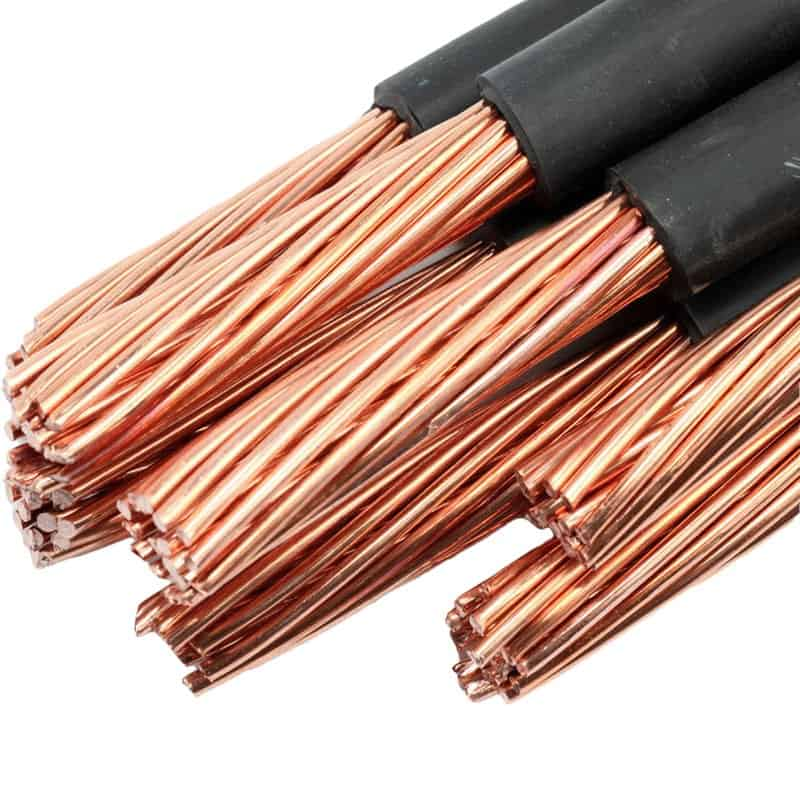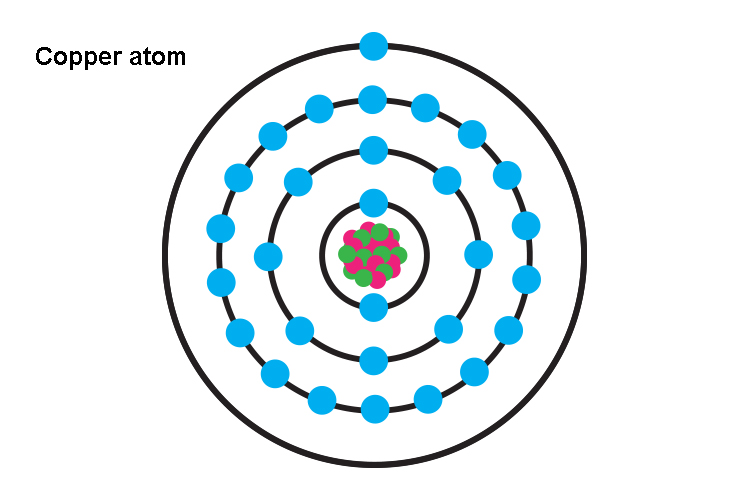# Does Copper Conduct Electricity?Copper is a metal element and is denoted by the symbol Cu. It has the atomic number 29. Like other metals, it is also malleable and ductile.

It is a reddish-orange element that is found in the free state of nature. It was the first metal to be used replacing stones by the Neolithic humans. A few important ores of copper are chalcocite, chalcopyrite, malachite, cuprite, etc.

So, does copper conduct electricity? Yes, copper is a conductor of electricity due to the presence of free charge carriers viz. electrons that allow the flow of current through it. The electronic configuration of copper is [Ar] 3d104s1. The valence electron in the 4s orbital is located far away from the nucleus and is, therefore, very loosely bound. When voltage is applied the valence electrons from the copper atoms dissociate and start moving from negative to the positive terminal and thus, conduct electricity.

Let us read out about the electrical conductance of copper in more detail.

Contents

## Is Copper a Good Conductor of Electricity?

Yes, copper is a good conductor of electricity. In fact, it is the best electrical conductor due to its high conductance and low resistivity value.

It is the most conductive metal after silver and this is the reason why it is the most frequently used material in various electrical appliances.

There is a certain amount of energy for every metal which when applied to its atom results in the increase of the kinetic energy of its valence electrons.Usually, nature provides this energy in the form of heat, and if a sufficient amount of energy is applied continuously to an atom, after some time the kinetic energy of its electrons becomes so large that it gets difficult for the valence electron to continue moving in their defined orbit due to which their orbital radius keeps expanding and ultimately the electron leaves their orbital and start moving freely in space.

These electrons move randomly inside the metal and thus, do not conduct electricity unless an electrical potential is applied.

As soon as the voltage is applied the randomly moving electrons get aligned in one direction and start moving from the negative to the positive terminal.

This directional movement of free or delocalized electrons is responsible for the conduction of electricity and the ease of their availability determines whether or not a substance can behave as a conductor or how good its conductance power is.

## Why is Copper a Good Conductor of Electricity?

As discussed in the previous section the conduction of electricity in metal is due to the presence of free electrons.

This indicates that the conductivity of a metal depends upon the ease with which the free electrons can be made available in that metal.

In the case of copper, the ionization energy is 7.72 eV, which is very low meaning thereby that it is quite easy for a copper atom to lose its valence electrons and form Cu+ ions.

This is because the valence electron in the copper atom is located in a 4s subshell which is placed far away from its positively charged nucleus.As the distance is too large the positive charge of the nucleus is unable to maintain its hold on to the negatively charged electron of the valence shell which ultimately detaches from the copper atom and starts moving randomly in free space around the atom.

Regarding more detail about the copper atoms, you can refer to is copper a pure substance.

These electrons move randomly canceling their charges amongst themselves unless an electric potential is applied.

The application of voltage fixes the two ends of the metal as positive and negative, respectively.

The electrons that are negatively charged start moving towards the positive terminal of the voltage source. This conduction/ flow of charges is known as the electric current.

## Conductivity of CopperThe conductivity of a substance refers to the ease with which it allows the electric current to pass through it.

It is measured in Siemens per meter (S/m) or mho per meter. It is denoted by the symbol σ and calculated using the formula given below:

σ = J / E

where,

σ = electrical conductivity (S/m or mho/m)

J = current density (amps/m2)

E = electric field strength (volts/m)

As electric current is the movement of charges, therefore, for a substance to act as a conductor it must have free charge carriers available.

Metals conduct electricity through the movement of free electrons that are present in their free space or delocalized electrons.

Therefore, in the case of metals, the electrical conductivity depends upon the availability of these free electrons.

The easy availability of these electrons makes a substance better a conductor of electricity.

In copper, the free electrons are readily available as the valence electrons are located in 4s orbital that is positioned far away from the positively charged nucleus these are very loosely bound and therefore, effortlessly leave the atom.

These electrons are able to drift freely between the atoms and thus, make copper a very good conductor of electricity.

The value of electrical conductivity for copper is 59.6 X 106 which is quite high indicating that it is a very good conductor of electricity.

## Resistance of a Copper wire

Resistance is the obstruction faced by the electric current while flowing through a conductor. It is denoted by the symbol R.

The electrical resistance is the reason why a conductor heats up when it carries the current for some time.

As per ohm’s law, the resistance of a conductor is directly proportional to its potential difference and inversely proportional to the current flowing through the conductor. It is given by the formula:

R = V/I

Where R = Resistance

V = Potential difference

I = Current

The resistance of a conductor depends upon certain factors viz. cross-sectional area, length, temperature, and material of the conductor.

Based on these another formula for resistance is given as:

R = ρL/A

Where R = Resistance

ρ = Resistivity

L = length of conductor

A = Cross-sectional area of conductor

Resistivity is the opposite of conductivity.

It is actually the qualitative measurement of the ability of a conductor to resist the flow of current passing through it.

It is also referred to as specific resistance. It is given by the following formula:

ρ=E/J

Where ρ = Resistivity (Ω.m)

E = Electric field (V/m)

J = Current density (A/m2)

The value of resistance for a substance depends upon the variable factors and therefore, has to be calculated in any given situation.

Resistance is an extrinsic property. However, the resistivity of a substance depends upon temperature and therefore, is more or less the same. It is an intrinsic property of a substance.

The value of resistivity for copper is 1.68×10−8 at 20 °C which is very low making copper a good conductor of electricity.

## Does the conductivity of Copper vary with Temperature?

Yes, the temperature variation affects the conductivity of copper which decreases with an increase in temperature.

Actually, the conductivity of copper is due to the movement of free electrons inside the metal which gain direction when an electric potential is applied and thus, help conduct current.

To some extent, the increase in temperature is responsible for increasing the kinetic energy of these electrons and helps them move out of the atoms into free space.

However, as the temperature further increases, it causes the movement of atoms inside the metal due to a great increase in their kinetic energy.

This atomic movement causes the lattice vibration which increases with temperature.

These vibrations cause the free electrons to collide with the moving atoms or the crystal lattice thereby hindering their path of electrons. As the electrons are unable to move freely it results in the decrease in conductivity of copper.## Properties of Copper

A few important properties of copper are:

• It is a reddish-orange color substance denoted by the symbol Cu

• The atomic mass of copper is 63.546.

• The melting and boiling points of copper are 1084.62 °C and 2562 °C, respectively.

• The density of copper is 8.96 g/cm3.

• It does not react with water but oxides in presence of atmospheric oxygen and forms copper oxide.

## Uses of Copper

Some of the uses of copper are listed below:

• Being a good conductor of electricity copper is used in making electrical cables and wires.

• Due to its high electrical conductivity and heat dissipation properties, it is used in making integrated circuits and heat sinks, respectively.

• Being corrosion-resistant copper is also used as a weatherproof architectural material.

• It is also used as an anti-biofouling and antimicrobial substance.

• It is used in making jewelry and compression clothing.

• Copper is also responsible for electron and oxygen transport reactions inside the body and thus, forms an essential trace element for most plants and animals.

## Conclusion

Copper conducts electricity owing to the presence of loosely bound valence electron that easily dissociates from their parent atom and moves in free space. Under the influence of an electric field, these free electrons from copper atoms help conduct electricity.

The outermost electron of copper is placed in the 4s sub-shell which is far away from the positively charged nucleus due to which the nucleus loses its grip and the electron easily moves out of the atom making copper a good conductor of electricity.

The electrical conductivity of copper is 59.6 X 106.

The electrical resistivity of copper is 1.68×10−8.

The electrical conductivity of copper decreases with the rise in temperature.